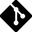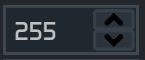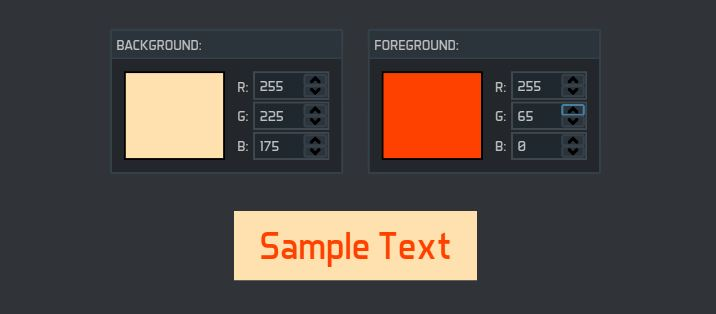# UserControl TutorialTutorial Data

No modern presentation framework would be complete without the ability to create your own reusable controls. If no existing control has a programmatic interface that naturally represents your concept, go ahead and create a user control or custom control.

UserControls can be seen as a composition of existing controls. They contain a logical tree defining its look and tend to have logic that directly interacts with these child elements.

In contrast CustomControls are needed when you want to create a totally new control or extend the functionality of an existing control. A custom control tends to get its look from a visual tree defined in a separate control template and generally has logic that works even if the user changes its visual tree completely.

In this tutorial we will focus on the development of a simple user control that implements the typical numeric spinner. The next tutorial will be dedicated to custom controls.

# Interface Creation

Let's create a very simple user control, a NumericUpDown control composed of two RepeatButtons to increment and decrement the value and a TextBlock to display it.NumericUpDown.xaml
```<UserControl
xmlns="http://schemas.microsoft.com/winfx/2006/xaml/presentation"
xmlns:x="http://schemas.microsoft.com/winfx/2006/xaml"
x:Class="UserControl.NumericUpDown">

<Grid>
<Grid.RowDefinitions>
<RowDefinition/>
<RowDefinition/>
</Grid.RowDefinitions>
<Grid.ColumnDefinitions>
<ColumnDefinition/>
<ColumnDefinition Width="Auto"/>
</Grid.ColumnDefinitions>

<Border Grid.RowSpan="2" Grid.ColumnSpan="2" BorderThickness="1"
BorderBrush="#40484F" Background="#20282F"/>

<TextBlock Grid.RowSpan="2" VerticalAlignment="Center" Margin="5,3,4,3" />

<RepeatButton Name="UpButton" Grid.Column="1" Grid.Row="0" Padding="4,1" Margin="0,2,2,0">
<Path Data="M1,1L4,4 7,1" Stroke="Black" StrokeThickness="2"
StrokeStartLineCap="Round" StrokeEndLineCap="Round" RenderTransformOrigin="0.5,0.5">
<Path.RenderTransform>
<ScaleTransform ScaleY="-1"/>
</Path.RenderTransform>
</Path>
</RepeatButton>

<RepeatButton Name="DownButton" Grid.Column="1" Grid.Row="1" Padding="4,1" Margin="0,0,2,2">
<Path Data="M1,1L4,4 7,1" Stroke="Black" StrokeThickness="2"
StrokeStartLineCap="Round" StrokeEndLineCap="Round"/>
</RepeatButton>

</Grid>

</UserControl>
```

This interface will correspond to a C++ class that implements the control's code-behind:

NumericUpDown.xaml.cpp
```class NumericUpDown: public UserControl
{
public:
NumericUpDown()
{
InitializeComponent();
}

private:
void InitializeComponent()
{
}

NS_IMPLEMENT_INLINE_REFLECTION(NumericUpDown, UserControl)
{
NsMeta<TypeId>("UserControl.NumericUpDown");
}
};
```

We are following the steps in the tutorial that describes how to extend NoesisGUI. The unique new detail here, apart from deriving from UserControl, is the GUI::LoadComponent call that indicates the XAML file that will be loaded when this user control is created.

# Properties

Now we define the properties that this control will expose. We talked about the numeric spinner value, so this could be our first property: Value. A dependency property must be declared as a public static member with getter and setter accessors to facilitate the use of the control within the code:

NumericUpDown.xaml.cpp
```class NumericUpDown: public UserControl
{
public:
NumericUpDown()
{
InitializeComponent();
}

int GetValue() const
{
return DependencyObject::GetValue<int>(ValueProperty);
}

void SetValue(int value)
{
DependencyObject::SetValue<int>(ValueProperty, value);
}

static const DependencyProperty* ValueProperty;

private:
void InitializeComponent()
{
}

NS_IMPLEMENT_INLINE_REFLECTION(NumericUpDown, UserControl)
{
NsMeta<TypeId>("UserControl.NumericUpDown");

UIElementData* data = NsMeta<UIElementData>(TypeOf<SelfClass>());
data->RegisterProperty<int>(ValueProperty, "Value",
}
};

const DependencyProperty* NumericUpDown::ValueProperty;
```

The interface has a text block to show the spinner value. If we want to automatically update the interface we can bind its Text property to the Value property we just created. Besides that, the interface has two buttons that increment or decrement the value of the spinner, so we can add some code-behind that responds to the Click event of these buttons to update the Value property appropriately:

NumericUpDown.xaml
```<UserControl
xmlns="http://schemas.microsoft.com/winfx/2006/xaml/presentation"
xmlns:x="http://schemas.microsoft.com/winfx/2006/xaml"
x:Class="UserControl.NumericUpDown"
x:Name="NumericUpDownControl">

<Grid>
<Grid.RowDefinitions>
<RowDefinition/>
<RowDefinition/>
</Grid.RowDefinitions>
<Grid.ColumnDefinitions>
<ColumnDefinition/>
<ColumnDefinition Width="Auto"/>
</Grid.ColumnDefinitions>

<Border Grid.RowSpan="2" Grid.ColumnSpan="2" BorderThickness="1"
BorderBrush="#40484F" Background="#20282F"/>

<TextBlock Grid.RowSpan="2" VerticalAlignment="Center" Margin="5,3,4,3"
Text="{Binding Value, ElementName=NumericUpDownControl}"/>

Margin="0,2,2,0" Click="UpButton_Click">
<Path Data="M1,1L4,4 7,1" Stroke="Black" StrokeThickness="2"
StrokeStartLineCap="Round" StrokeEndLineCap="Round" RenderTransformOrigin="0.5,0.5">
<Path.RenderTransform>
<ScaleTransform ScaleY="-1"/>
</Path.RenderTransform>
</Path>
</RepeatButton>

Margin="0,0,2,2" Click="DownButton_Click">
<Path Data="M1,1L4,4 7,1" Stroke="Black" StrokeThickness="2"
StrokeStartLineCap="Round" StrokeEndLineCap="Round"/>
</RepeatButton>

</Grid>

</UserControl>
```
NumericUpDown.xaml.cpp
```class NumericUpDown: public UserControl
{
public:
NumericUpDown()
{
InitializeComponent();
}

int GetValue() const
{
return DependencyObject::GetValue<int>(ValueProperty);
}

void SetValue(int value)
{
DependencyObject::SetValue<int>(ValueProperty, value);
}

static const DependencyProperty* ValueProperty;

private:
void InitializeComponent()
{
}

bool ConnectEvent(BaseComponent* source, const char* event, const char* handler) override
{
NS_CONNECT_EVENT(Button, Click, UpButton_Click);
NS_CONNECT_EVENT(Button, Click, DownButton_Click);
return false;
}

void UpButton_Click(BaseComponent*, const Noesis::RoutedEventArgs&)
{
int step = DependencyObject::GetValue<int>(StepValueProperty);
SetValue(GetValue() + step);
}

void DownButton_Click(BaseComponent*, const Noesis::RoutedEventArgs&)
{
int step = DependencyObject::GetValue<int>(StepValueProperty);
SetValue(GetValue() - step);
}

NS_IMPLEMENT_INLINE_REFLECTION(NumericUpDown, UserControl)
{
NsMeta<TypeId>("UserControl.NumericUpDown");

UIElementData* data = NsMeta<UIElementData>(TypeOf<SelfClass>());
data->RegisterProperty<int>(ValueProperty, "Value",
}
};

const DependencyProperty* NumericUpDown::ValueProperty;
```

To connect events with code-behind functions we override ConnectEvent virtual function. This function is called for each event referenced in the associated xaml, and provides the event source along with the name of function that is expected to be called.

# Events

Next step is exposing an event to notify users when the value of the numeric spinner changes. We call this event ValueChanged and implement it as a routed event. Routed events must be declared with a public static member and with a virtual function that raises the event so inheritors can override the basic implementation:

NumericUpDown.xaml.cpp
```class NumericUpDown: public UserControl
{
public:
NumericUpDown()
{
InitializeComponent();
}

int GetValue() const
{
return DependencyObject::GetValue<int>(ValueProperty);
}

void SetValue(int value)
{
DependencyObject::SetValue<int>(ValueProperty, value);
}

/// Occurs when numeric value changes
RoutedEvent_<RoutedPropertyChangedEventHandler<int>::Handler> ValueChanged()
{
return RoutedEvent_<RoutedPropertyChangedEventHandler<int>::Handler>(this, ValueChangedEvent);
}

static const DependencyProperty* ValueProperty;
static const RoutedEvent* ValueChangedEvent;

protected:
virtual void OnValueChanged(const RoutedPropertyChangedEventArgs<int>& args)
{
RaiseEvent(args);
}

private:
void InitializeComponent()
{
}

bool ConnectEvent(BaseComponent* source, const char* event, const char* handler) override
{
NS_CONNECT_EVENT(Button, Click, UpButton_Click);
NS_CONNECT_EVENT(Button, Click, DownButton_Click);
return false;
}

void UpButton_Click(BaseComponent*, const Noesis::RoutedEventArgs&)
{
int step = DependencyObject::GetValue<int>(StepValueProperty);
SetValue(GetValue() + step);
}

void DownButton_Click(BaseComponent*, const Noesis::RoutedEventArgs&)
{
int step = DependencyObject::GetValue<int>(StepValueProperty);
SetValue(GetValue() - step);
}

static void OnValueChangedStatic(DependencyObject* d,
const DependencyPropertyChangedEventArgs& e)
{
NumericUpDown* control = static_cast<NumericUpDown*>(d);
int oldValue = *static_cast<const int*>(args.oldValue);
int newValue = *static_cast<const int*>(args.newValue);

RoutedPropertyChangedEventArgs<int> e(control, ValueChangedEvent, oldValue, newValue);
control->OnValueChanged(e);
}

NS_IMPLEMENT_INLINE_REFLECTION(NumericUpDown, UserControl)
{
NsMeta<TypeId>("UserControl.NumericUpDown");

Ptr<UIElementData> data = NsMeta<UIElementData>(TypeOf<SelfClass>());
data->RegisterProperty<int>(ValueProperty, "Value",

data->RegisterEvent(ValueChangedEvent, "ValueChanged", RoutingStrategy_Bubbling);
}
};

const DependencyProperty* NumericUpDown::ValueProperty;
const RoutedEvent* NumericUpDown::ValueChangedEvent;
```

# Improvements

The source code that accompanies this tutorial incorporates several improvements. We have extended the functionality of the spinner by adding new dependency properties to control the maximum and minimum value, and the step factor whenever the up/down buttons are clicked. It is recommended that you read the code carefully.

# Usage

Now we can use our control in any other XAML file. For example as an editor for the values of an RGB color:```<Grid
xmlns="http://schemas.microsoft.com/winfx/2006/xaml/presentation"
xmlns:x="http://schemas.microsoft.com/winfx/2006/xaml"
UseLayoutRounding="True">

<StackPanel Orientation="Horizontal" HorizontalAlignment="Center" VerticalAlignment="Center">
<Grid>
<Grid.ColumnDefinitions>
<ColumnDefinition/>
<ColumnDefinition Width="Auto"/>
<ColumnDefinition/>
</Grid.ColumnDefinitions>

<Rectangle Stretch="Fill" Stroke="Black" Width="80">
<Rectangle.Fill>
<SolidColorBrush x:Name="BgColor" Color="White"/>
</Rectangle.Fill>
</Rectangle>

<Grid Grid.Column="1" Margin="10,0,4,0">
<Grid.RowDefinitions>
<RowDefinition/>
<RowDefinition/>
<RowDefinition/>
</Grid.RowDefinitions>
<TextBlock Text="R:" HorizontalAlignment="Right" VerticalAlignment="Center" Grid.Row="0"/>
<TextBlock Text="G:" HorizontalAlignment="Right" VerticalAlignment="Center" Grid.Row="1"/>
<TextBlock Text="B:" HorizontalAlignment="Right" VerticalAlignment="Center" Grid.Row="2"/>
</Grid>

<Grid Grid.Column="2" Width="60">
<Grid.RowDefinitions>
<RowDefinition/>
<RowDefinition/>
<RowDefinition/>
</Grid.RowDefinitions>
<NumericUpDown Grid.Row="0" Margin="0,0,0,0"/>
<NumericUpDown Grid.Row="1" Margin="0,2,0,0"/>
<NumericUpDown Grid.Row="2" Margin="0,2,0,0"/>
</Grid>

</Grid>
</GroupBox>
<Grid>
<Grid.ColumnDefinitions>
<ColumnDefinition/>
<ColumnDefinition Width="Auto"/>
<ColumnDefinition/>
</Grid.ColumnDefinitions>

<Rectangle Stretch="Fill" Stroke="Black" Width="80">
<Rectangle.Fill>
<SolidColorBrush x:Name="FgColor" Color="Black"/>
</Rectangle.Fill>
</Rectangle>

<Grid Grid.Column="1" Margin="10,0,4,0">
<Grid.RowDefinitions>
<RowDefinition/>
<RowDefinition/>
<RowDefinition/>
</Grid.RowDefinitions>
<TextBlock Text="R:" HorizontalAlignment="Right" VerticalAlignment="Center" Grid.Row="0"/>
<TextBlock Text="G:" HorizontalAlignment="Right" VerticalAlignment="Center" Grid.Row="1"/>
<TextBlock Text="B:" HorizontalAlignment="Right" VerticalAlignment="Center" Grid.Row="2"/>
</Grid>

<Grid Grid.Column="2" Width="60">
<Grid.RowDefinitions>
<RowDefinition/>
<RowDefinition/>
<RowDefinition/>
</Grid.RowDefinitions>
<NumericUpDown Grid.Row="0" Margin="0,0,0,0"/>
<NumericUpDown Grid.Row="1" Margin="0,2,0,0"/>
<NumericUpDown Grid.Row="2" Margin="0,2,0,0"/>
</Grid>

</Grid>
</GroupBox>
<TextBlock Text="Sample Text" HorizontalAlignment="Center" Margin="20,30,0,0" FontSize="24" Padding="10,5"
VerticalAlignment="Center" Background="{Binding ElementName=BgColor}"
Foreground="{Binding ElementName=FgColor}"/>
</StackPanel>
</Grid>
```